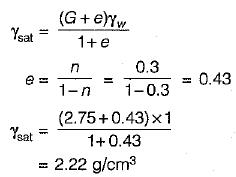Courses

# Test: Properties of Soil - 3

## 10 Questions MCQ Test Topicwise Question Bank for Civil Engineering | Test: Properties of Soil - 3

Description
This mock test of Test: Properties of Soil - 3 for Civil Engineering (CE) helps you for every Civil Engineering (CE) entrance exam. This contains 10 Multiple Choice Questions for Civil Engineering (CE) Test: Properties of Soil - 3 (mcq) to study with solutions a complete question bank. The solved questions answers in this Test: Properties of Soil - 3 quiz give you a good mix of easy questions and tough questions. Civil Engineering (CE) students definitely take this Test: Properties of Soil - 3 exercise for a better result in the exam. You can find other Test: Properties of Soil - 3 extra questions, long questions & short questions for Civil Engineering (CE) on EduRev as well by searching above.
QUESTION: 1

### Select the correct range of Density Index ID

Solution: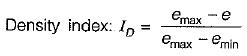e can range from emax to emin depending upon loosest condition to densest condition.

QUESTION: 2

### If the plasticity index of a soil mass is zero the soil is

Solution:

Coarse grained soils like sand cannot achieve the plastic state of consistency and their liquid and plastic limit are said to coincide.

QUESTION: 3

### The ratio of the shear strength of undisturbed soil to that of remoulded soil is known as the

Solution:
QUESTION: 4

Stake’s law is valid only if the size of the particle is

Solution:

Diameter larger than 0.2 mm causes turbulence.
Diameter less than 0.0002 mm causes brownian movement and velocity of settlement is too small for accurate measurement.

QUESTION: 5

Water content of soil can be

Solution:

The water content of some of the fine-gained soils may be even more than 100%, which indicates that more than 50% of the total mass is that of water.

QUESTION: 6

Relative density of a compacted dense sand is approximately equal to

Solution: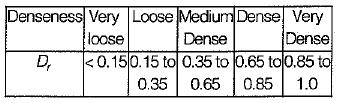QUESTION: 7

If the volume of voids is equal to the volume of solids in a soil mass, then the values of porosity and void ratio are

Solution: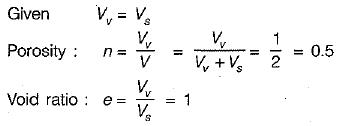QUESTION: 8

The submerged density of soil in terms of unit wt. of water Yw; specific gravity G and void ratio e is given by the. expression

Solution:
QUESTION: 9

A soil has plastic limit of 20% and plasticity index of 40%. if the natural water content of the soil is 34%, then the consistency index is equal to

Solution: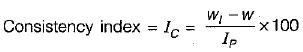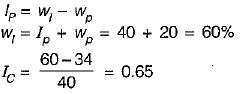QUESTION: 10

The porosity of a soil sample is 30% and specific gravity of its particles is 2.75. Then Saturated unit weight is equal to

Solution: